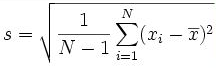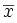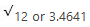Altair® Panopticon

# Stdev

The Standard Deviation of the selection.

The Standard Deviation is a measure of how spread out numbers are in a set. The Deviation just means how far from the normal.

Stdev is used when the group of numbers being evaluated is only a partial sampling of the whole population.

The formula:Whereis the mean computed by getting the sum of all the items and dividing them by the number of items minus one.

Sample 1:

Given a set of numbers like 12, 6, 12.

## Computing the Stdev

1. Compute the mean of the sample.

Mean = (Sum of items/n), where n is the number of items

12+6+12/3=10

1. Square the difference between each point and the mean

(12-10)^2 =4

(6-10)^2 =16

(12-10)^2 =4

1. Calculate the average of the results in step 2 above

4+16+4/3-1=24/2

1. Compute the square root of the result in step 4.Sample 2:

Given the following sample fields:

 Number Arbitrary Negative Values Positive Values One Binary Currency Decimal 1 3 -1 1 1 0 \$1.00 1.01 2 2 -2 2 1 \$10.00 2.02 3 3 -3 3 0 \$100.00 3.03 4 0 -4 4 1 \$1,000.00 4.04 5 -1 -5 5 0 \$10,000.00 5.05 6 -2 -6 6 1 -\$1.00 6.06 7 -3 -7 7 0 -\$10.00 7.07 8 0 -8 8 -\$100.00 8.08 9 0 -9 9 -\$1,000.00 9.09 10 0 -10 0 \$0.00 0.00

Sample fields

The Stdev for each field:

 Number Arbitrary Negative Values Positive Values One Binary Currency Decimal 3.0277 1.7638 3.0277 3.0277 0 .5345 \$3,197.5720 3.0579

Results per field